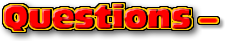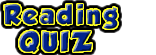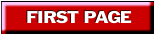ChaptersThis text is meant to accompany class discussions. It is not everything there is to know about the basics of electricity. It is meant as a  prep for class. More detailed notes and examples are given in the class notes, presentations, and demonstrations (click here.)Click for the questions that go with this reading
Subtracting Vectors

In math class we are taught the the negative sign means the "opposite." With vectors we now know that the negative sign means, "the opposite direction." Therefore in a math equation,, means the opposite direction of. Now formulas and equation mean more than just adding and removing apples from a basket. By putting vectors in equations, the quantities have direction. Math equations that are written with vectors can be solved with a picture. For example:

In this example, the answer "R" is shows us its direction is 2 blocks to the right and up 1 block. Its magnitude is approximately 1.7 blocks long. If each block represents a meter then the magnitude is 1.7 meters. Did you notice how the "A" vector change to the opposite of "A" vector? Run again and watch the "A" vector. To summarize what is going on, subtracting vectors is adding the opposite, (direction).by Tony Wayne ...(If you are a teacher, please feel free to use these resources in your teaching.)